Miscellaneous

Chapter 9 Class 11 Sequences and Series
Serial order wise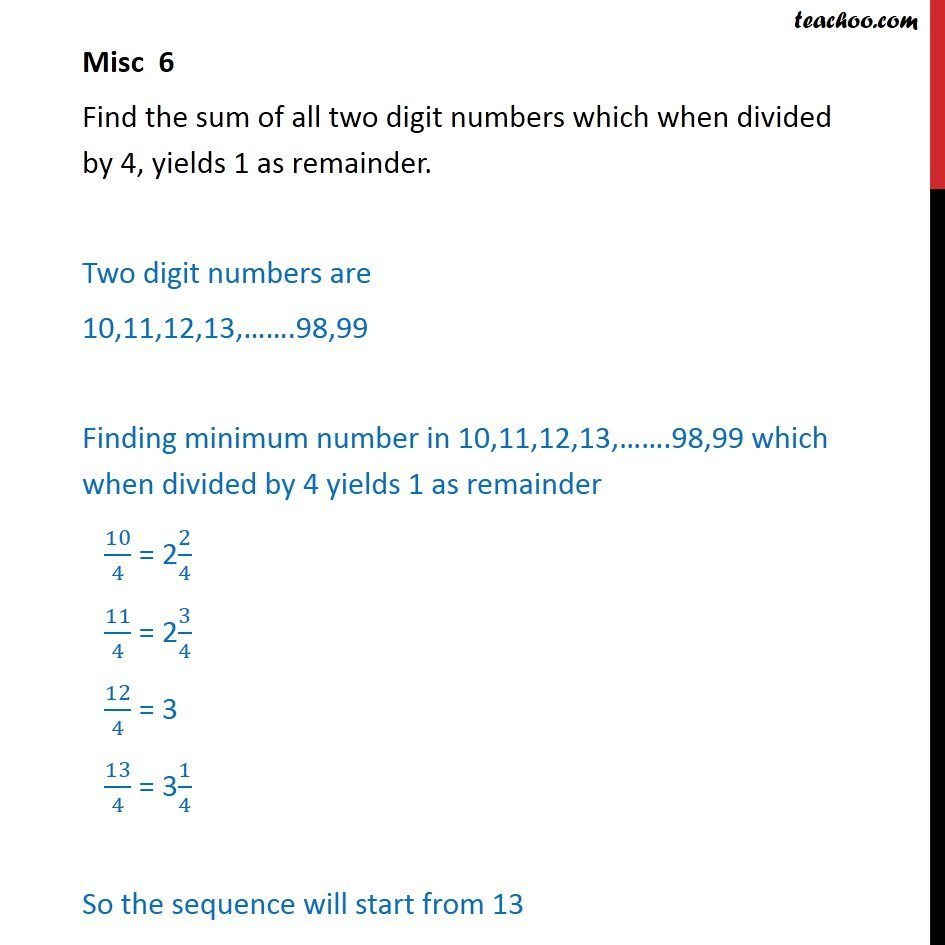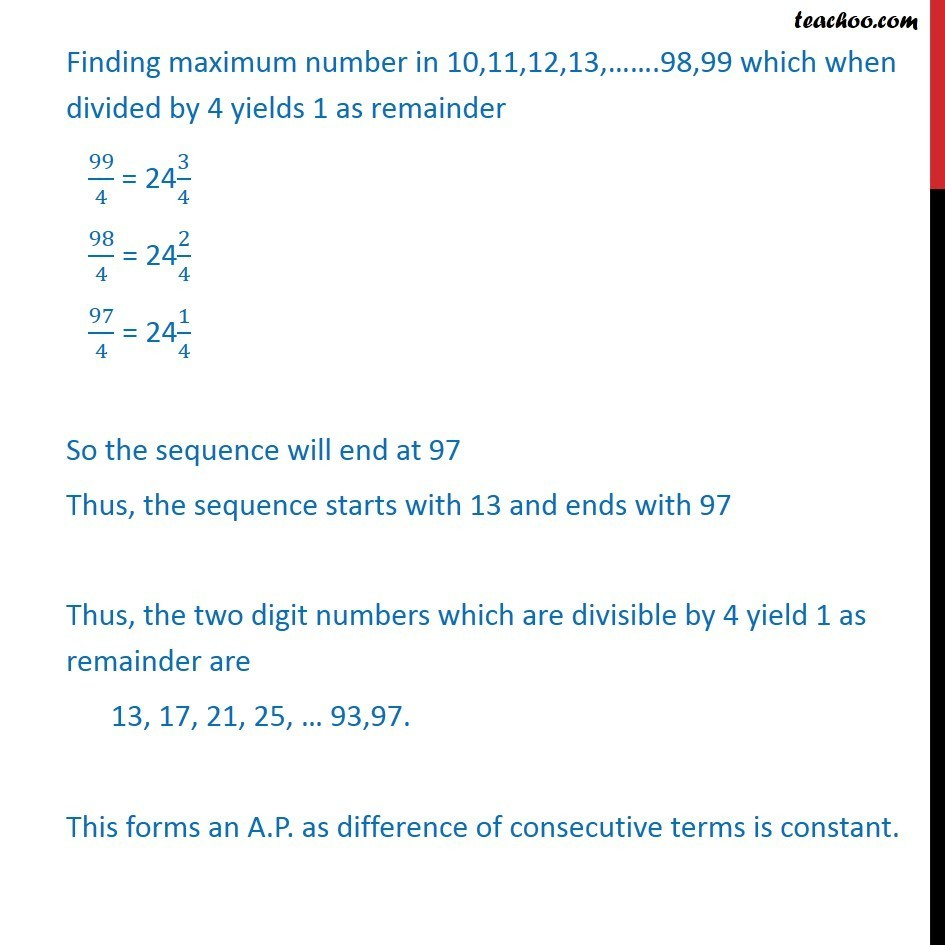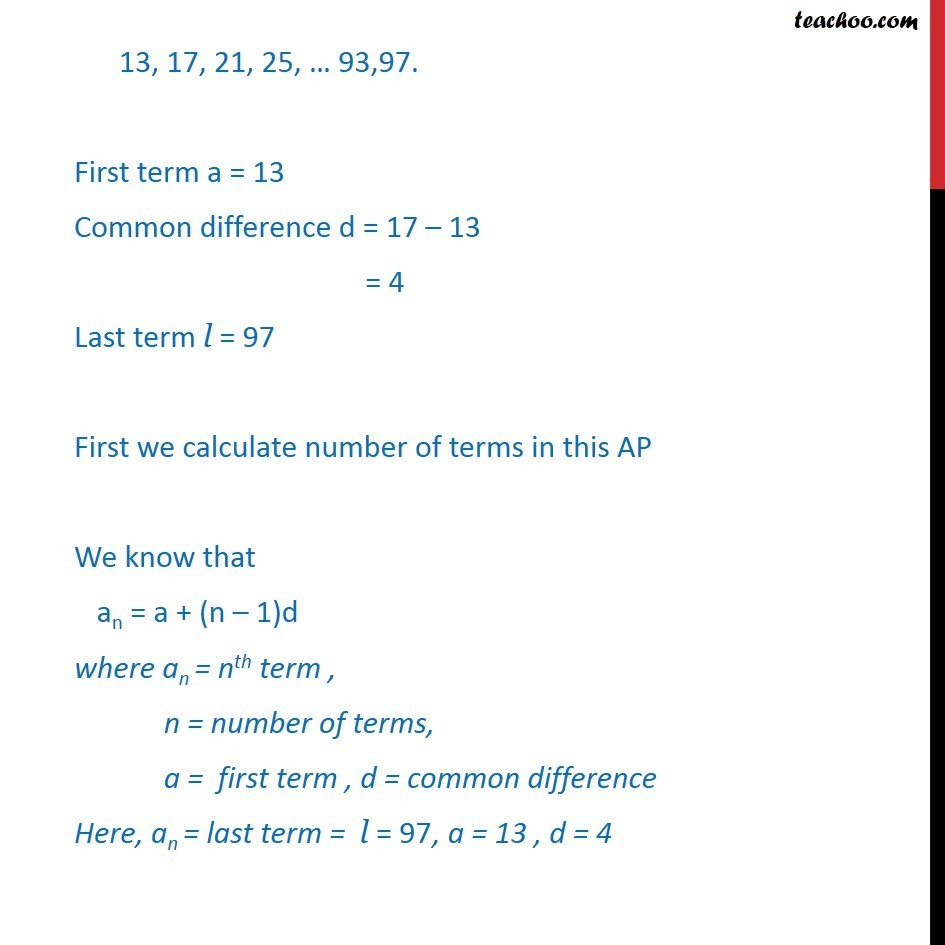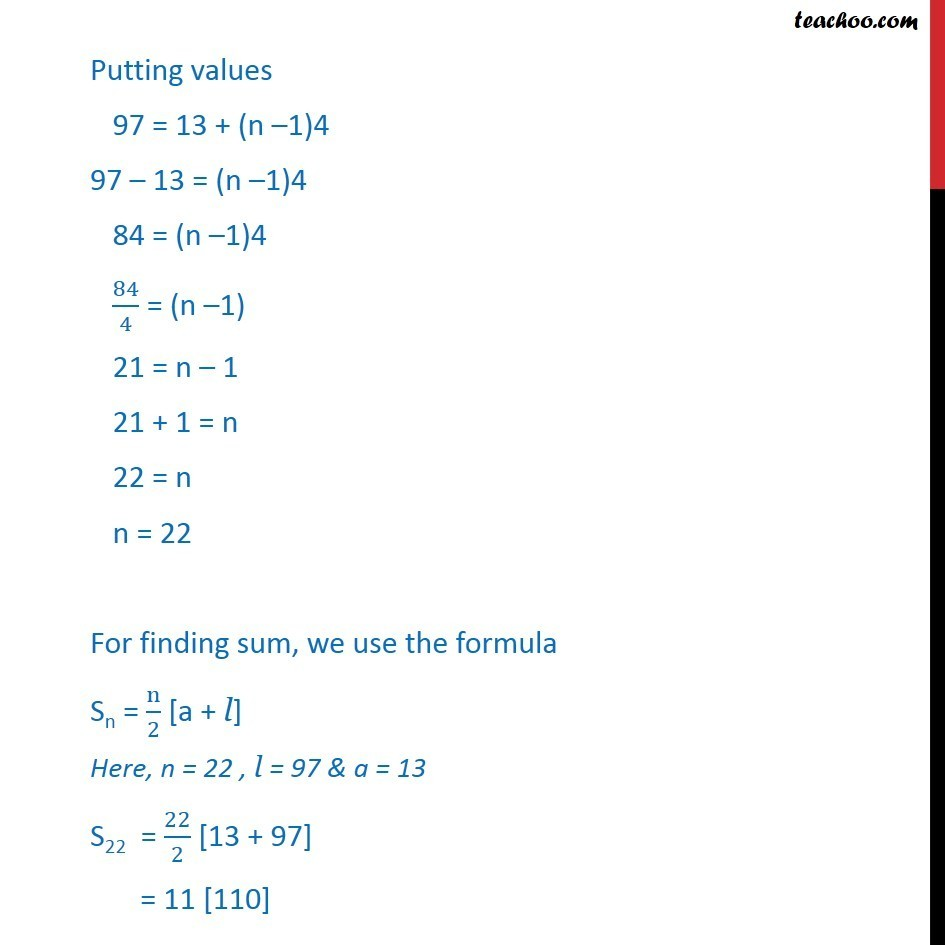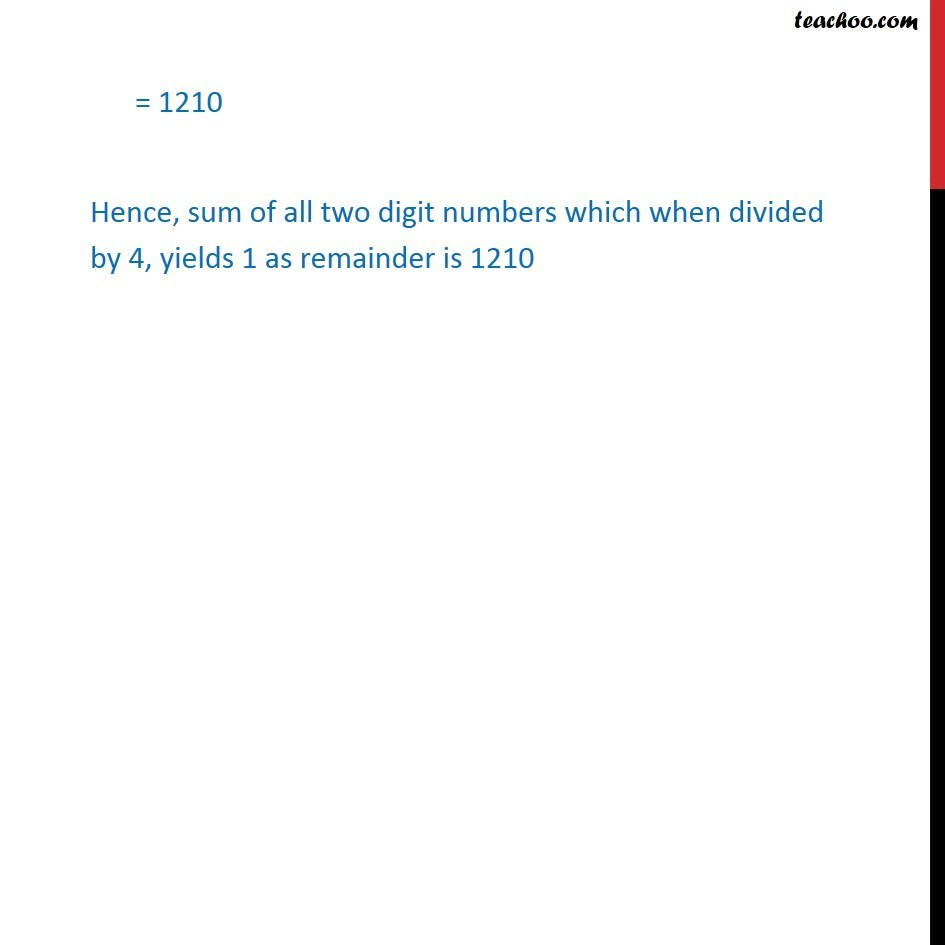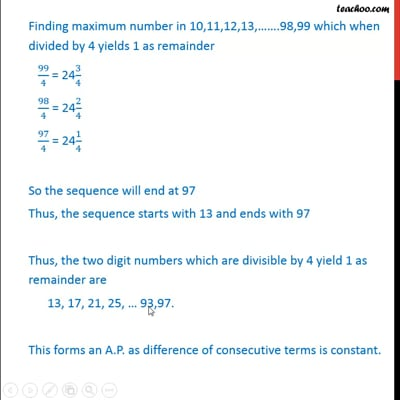This video is only available for Teachoo black users

Solve all your doubts with Teachoo Black (new monthly pack available now!)

### Transcript

Misc 6 Find the sum of all two digit numbers which when divided by 4, yields 1 as remainder. Two digit numbers are 10,11,12,13, .98,99 Finding minimum number in 10,11,12,13, .98,99 which when divided by 4 yields 1 as remainder 10/4 = 22/4 11/4 = 23/4 12/4 = 3 13/4 = 31/4 So the sequence will start from 13 Finding maximum number in 10,11,12,13, .98,99 which when divided by 4 yields 1 as remainder 99/4 = 243/4 98/4 = 242/4 97/4 = 241/4 So the sequence will end at 97 Thus, the sequence starts with 13 and ends with 97 Thus, the two digit numbers which are divisible by 4 yield 1 as remainder are 13, 17, 21, 25, 93,97. This forms an A.P. as difference of consecutive terms is constant. 13, 17, 21, 25, 93,97. First term a = 13 Common difference d = 17 13 = 4 Last term l = 97 First we calculate number of terms in this AP We know that an = a + (n 1)d where an = nth term , n = number of terms, a = first term , d = common difference Here, an = last term = l = 97 , a = 13 , d = 4 Putting values 97 = 13 + (n 1)4 97 13 = (n 1)4 84 = (n 1)4 84/4 = (n 1) 21 = n 1 21 + 1 = n 22 = n n = 22 For finding sum, we use the formula Sn = n/2 [a + l] Here, n = 22 , l = 97 & a = 13 S22 = 22/2 [13 + 97] = 11  = 1210 Hence, sum of all two digit numbers which when divided by 4, yields 1 as remainder is 1210